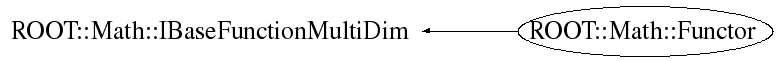# class ROOT::Math::Functor: public ROOT::Math::IBaseFunctionMultiDim

```
Documentation for class Functor class.
It is used to wrap in a very simple and convenient way multi-dimensional function objects.
It can wrap all the following types:
<ul>
<li> any C++ callable object implemention double operator()( const double *  )
<li> a free C function of type double ()(double * )
<li> a member function with the correct signature like Foo::Eval(const double * ).
In this case one pass the object pointer and a pointer to the member function (&Foo::Eval)
</ul>
The function dimension is required when constructing the functor.

@ingroup  CppFunctions

```

## Function Members (Methods)

public:
 virtual ~Functor() virtual ROOT::Math::Functor::ImplBase* Clone() const ROOT::Math::Functor Functor() ROOT::Math::Functor Functor(const ROOT::Math::Functor&) ROOT::Math::Functor Functor(void* p, unsigned int dim, const char* className = 0, const char* methodName = 0) virtual unsigned int NDim() const double ROOT::Math::IBaseFunctionMultiDim::operator()(const double* x) const ROOT::Math::Functor& operator=(const ROOT::Math::Functor& rhs)
private:
 virtual double DoEval(const double* x) const

## Data Members

private:
 auto_ptr fImpl pointer to base functor handler

## Class Charts## Function documentation

BaseFunc * Clone()
``` clone of the function handler (use copy-ctor)
```
unsigned int NDim()
``` constructor for multi-dimensional functions
```
double DoEval(const double* x) const
Functor(const ROOT::Math::Functor& )
```Default constructor

```
`{}`
Functor(const PtrObj& p, MemFn memFn, unsigned int dim )
```construct from a pointer to member function (multi-dim type)

```
`{}`
Functor( const Func & f, unsigned int dim )
```construct from a callable object of multi-dimension
with the right signature (implementing operator()(double *x)

```
`{}`
virtual ~Functor()
```Destructor (no operations)

```
`{}`

Author: L. Moneta Mon Nov 13 15:58:13 2006
Last update: root/mathcore:\$Id: Functor.h 21553 2007-12-21 10:55:46Z moneta \$
Copyright (c) 2006 LCG ROOT Math Team, CERN/PH-SFT *

This page has been automatically generated. If you have any comments or suggestions about the page layout send a mail to ROOT support, or contact the developers with any questions or problems regarding ROOT.